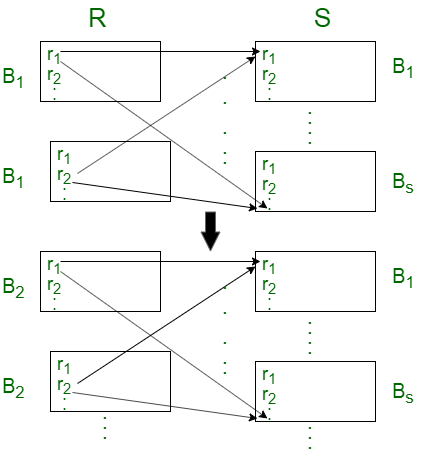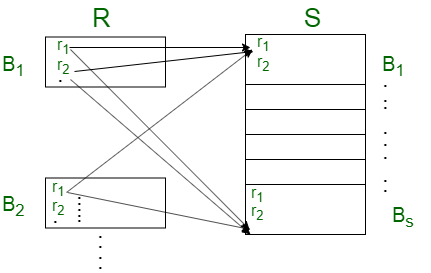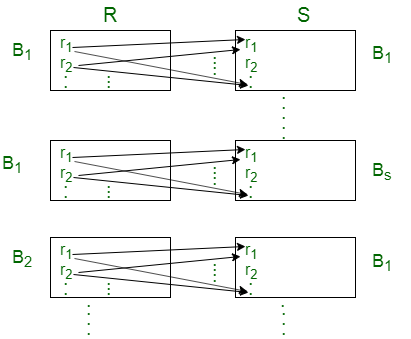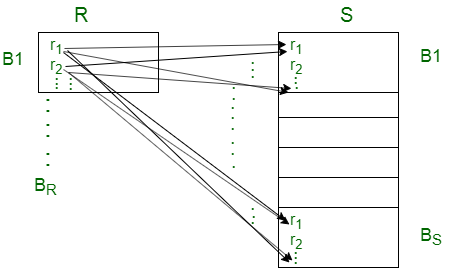GFG App
Open AppBrowser
Continue

# Join algorithms in Database

There are two algorithms to compute natural join and conditional join of two relations in database: Nested loop join, and Block nested loop join.

To understand these algorithms we will assume there are two relations, relation R and relation S. Relation R has TR tuples and occupies BR blocks. Relation S has TS tuples and occupies BS blocks. We will also assume relation R is the outer relation and S is the inner relation.

### Nested Loop Join

In the nested loop join algorithm, for each tuple in outer relation, we have to compare it with all the tuples in the inner relation then only the next tuple of outer relation is considered. All pairs of tuples which satisfy the condition are added in the result of the join.

```for each tuple tR in TR do
for each tuple ts in Ts do
compare (tR, ts) if they satisfies the condition
add them in the result of the join
end
end```

This algorithm is called nested join because it consists of nested for loops.

Let’s see some cases to understand the performance of this algorithm,

Case-1: Assume only two blocks of main memory are available to store blocks from R and S relation.For each tuple in relation to R, we have to transfer all blocks of relation S and each block of relation R should be transferred only once.
So, the total block transfers needed = TR * BS + BR

Case-2: Assume one relation fits entirely in the main memory and there is at least space for one extra block.In this case, the blocks of relation S (that is, the inner relation) are only transferred once and kept in the main memory and the blocks of relation R are transferred sequentially. So, all the blocks of both the relation are transferred only once.
So, the total block transfers needed = BR + BS

The relation with a lesser number of blocks should be the outer relation to minimizing the total number of blocks access required in the main memory to complete the join.
That is, min(BR, BS)+1 is the minimum number of blocks in the main memory required to join two relations so that no block is transferred more than once.

In nested loop join, more access cost is required to join relations if the main memory space allocated for join is very limited.

### Block Nested Loop Join:

In block nested loop join, for a block of outer relation, all the tuples in that block are compared with all the tuples of the inner relation, then only the next block of outer relation is considered. All pairs of tuples which satisfy the condition are added in the result of the join.

```for each block bR in BR do
for each block bs in BS do
for each tuple tR in TR do
for each tuple ts in Ts do
compare (tR, ts) if they satisfies the condition
add them in the result of the join
end
end
end
end ```

Let’s look at some similar cases as nested loop join,

Case-1: Assume only two blocks of main memory are available to store blocks from R and S relation.For each block of relation R, we have to transfer all blocks of relation S and each block of relation R should be transferred only once.
So, the total block transfers needed = BR+ BR * BS

Case-2: Assume one relation fits entirely in the main memory and there is at least space for one extra block.In this case, total block transfers needed are similar to nested loop join.
Block nested loop join algorithm reduces the access cost compared to nested loop join if the main memory space allocated for join is limited.

Related GATE questions:

My Personal Notes arrow_drop_up